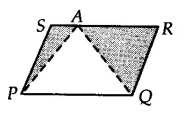Q

# A farmer was having a field in the form of a parallelogram PQRS. She took any point A on RS and joined it to points P and Q. In how many parts the fields is divided? What are the shapes of these parts?

Q: 6

A farmer was having a field in the form of a parallelogram PQRS. She took any point A on RS and joined it to points P and Q. In how many parts the fields is divided? What are the shapes of these parts? The farmer wants to sow wheat and pulses in equal portions of the field separately. How should she do it?

Views

We have a field in the form of parallelogram PQRS and a point A is on the side RS. Join AP and AQ. The field is divided into three parts i.e, APS, QAR and PAQ.Since APQ and parallelogram, PQRS is on the same base PQ and between same parallels RS and PQ.
Therefore, ............(i)
We can write above equation as,

ar (||gm PQRS) - [ar (APS) + ar(QAR)] = 1/2 .ar(PQRS)

from equation (i),

Hence, she can sow wheat in APQ and pulses in [APS + QAR] or wheat in [APS + QAR] and pulses in  APQ.

Exams
Articles
Questions